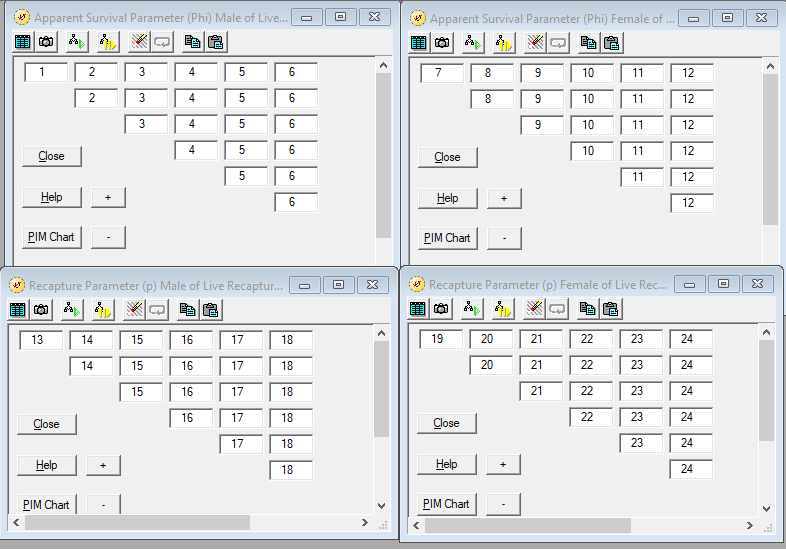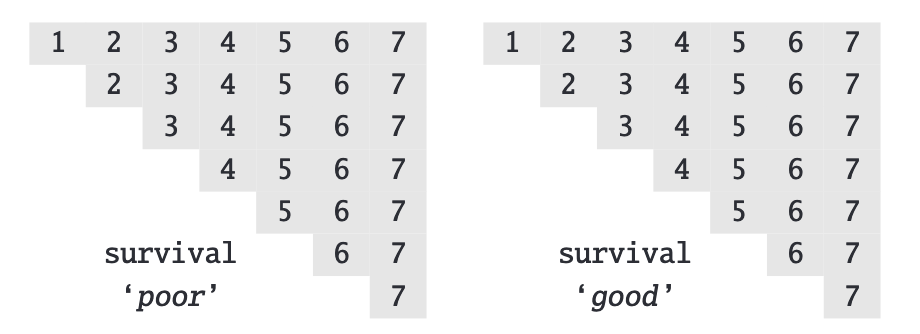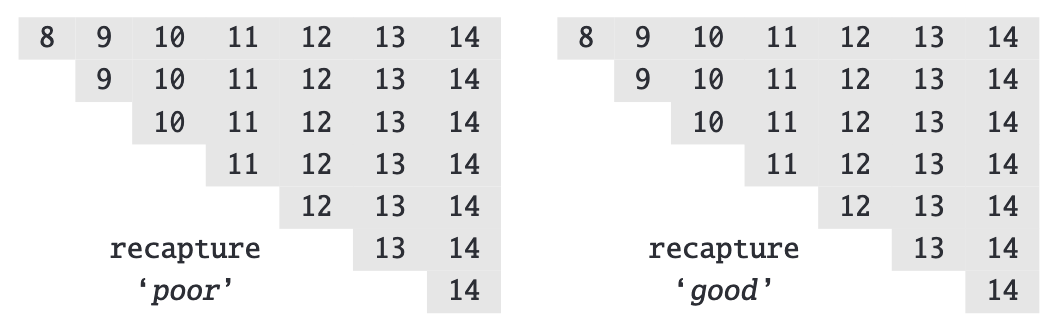You now have a bit of experience running models for live recaptures data in Program MARK. So far, you manipulated the Parameter Index Matrices (PIMs) to set up models of interest based on material in Chapter 3 of CW. Using the PIMs to set up models works fine for some model structures and is all that is needed for some problems. For example, you might be interested in running a model in which $$\phi_t$$ for years $$1, 2, \ldots , t$$ depends on (1) site type (managed or not) and (2) sex. Features like site type and sex are categorical and apply to many animals. Information on such covariates can be provided to MARK by grouping the input data (see Chapter 2 of CW) and used in modeling as shown in Chapters 3 and 4 of CW by working with PIM numbering.

PIMs

When you start a new analysis project in Program MARK, you indicate what type of data you’ll be working with and how many groups you’ll be entering data for. Entering data by group is one (but not the only) way of entering categorical covariate information into MARK. You will have 1 PIM for each parameter type for each group. For live recaptures data, if you enter data for 2 groups (male and female dippers) for a 7-year study, you will have a PIM for $$\phi_{male}$$, $$\phi_{female}$$, $$p_{male}$$, and $$p_{female}$$. Each PIM for this data type will be triangular and, by default, initially be numbered as shown below where each column of each PIM has it’s own unique number.Practice

Work your way through Page 1-24 of Chapter 4 of CW and try out the various techniques shown in Program MARK to model live recaptures data by renumbering the PIMs in useful ways. To help solidify your understanding, work with the input file AA.inp and fit the 6 models shown in the Results Browser on page 4-24 of Chapter 4 of CW.

Check on understanding

Which model is represented by the following PIMs for $$\phi$$ and $$p$$?